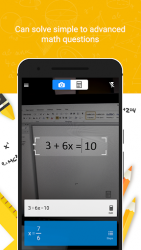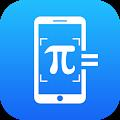##Photo Calculator - Smart Calculator and Math Solver   Version:  1.1

### Application Description:

Use your phone-s camera to solve equations with Smart Calculator- Math Calculator - Math Solver. Are you looking for quick and accurate math answers but not specific math calculator? With Photo Calculator, your phone can become Math scanner to solve math problem and immediately receive the mathway answers. Smart Calculator can simply snap photo calculator and math solver instantly delivers the mathway answers, math solution while also providing step by step solver. Math Solver is a valuable tool for a parent helping with his kid -s math homework and attempting to remember grade-school arithmetic or middle-school algebra, photo calculator. Math app can help students check their work and show them how to arrive at the correct math answers. And Math problem solver can help parents brush up on mathway lessons learned long ago. -Handwritten questions in Smart Calculator- Math solver app are currently not supported but they will be solved soon. Photo Calculator app calculates mathematics problems with camera.
Click a photo calculator, crop the portion and save it.
Math Problem Solver can solve simple as well as complex math problems.
Smart Calculator also contains manual math calculator.
Math Scanner with no internet required.
Step by step solutions for different methods to solve math problem.
Math Solver with Graphing and Tables, algebra calculator or math calculator.
Math Calculator can solve simple to advanced math questions.
Smart Calculator instants mathway solutions from algebra to trig and calculus.
Students will certainly use Math Calculator to do the heavy lifting for them.
The basic photo calculator in this Photo Calculator app contains add up, subtraction, multiplication and division.

## Conclusion

To conclude Photo Calculator - Smart Calculator and Math Solver works on Android operating system and can be easily downloaded using the below download link according to Freeware license. Photo Calculator - Smart Calculator and Math Solver download file is only 14.33 MB  in size.
Photo Calculator - Smart Calculator and Math Solver was filed under the Business category and was reviewed in softlookup.com and receive 5/5 Score.
Photo Calculator - Smart Calculator and Math Solver has been tested by our team against viruses, spyware, adware, trojan, backdoors and was found to be 100% clean. We will recheck Photo Calculator - Smart Calculator and Math Solver when updated to assure that it remains clean.

### Photo Calculator - Smart Calculator and Math Solver user Review

Please review Photo Calculator - Smart Calculator and Math Solver application and submit your comments below. We will collect all comments in an effort to determine whether the Photo Calculator - Smart Calculator and Math Solver software is reliable, perform as expected and deliver the promised features and functionalities.